# Basic Division Math Drill Worksheets

The division math facts worksheets created in this section help students master the instant recall of their division facts.

## Division facts review tables

We start this section with division facts review in table formats of 1-10, and 1-12. There are tables with answers and no answer. This table presents the subtraction facts 1-10 with answers: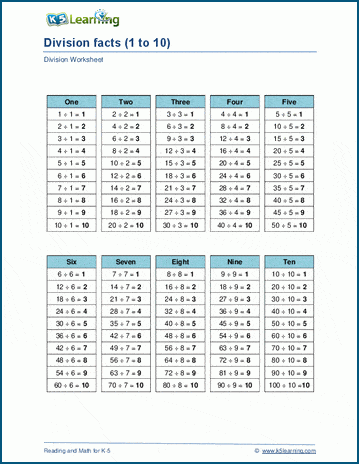## Division facts on the horizontal worksheets

There are three separate sets of worksheets in this area: division facts with small dividends, dividends up to 100 and dividends up to 144. The division facts with small dividends worksheets looks like this: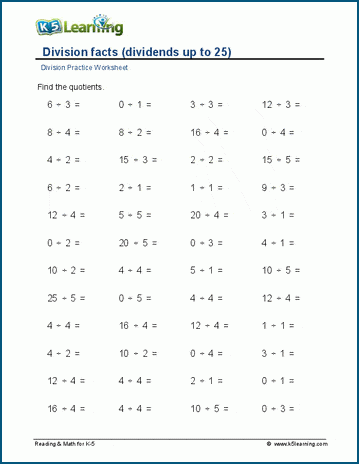## Worksheets covering long division

Now we move onto long division, starting with small dividends and working our way up to division facts to 100, and then 144. This is one of the worksheets covering division facts on the vertical to 100: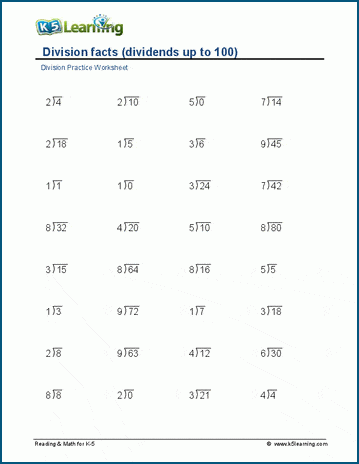## Fixed divisor math facts worksheets

We have separate pages of worksheets of dividing by 2, 3, 4, 5, 6, 7, 8, 9, 10, 11 and 12. Each page has separate worksheets for the number of quotients for different levels of difficulty. One of the the dividing by 6 worksheet looks like this: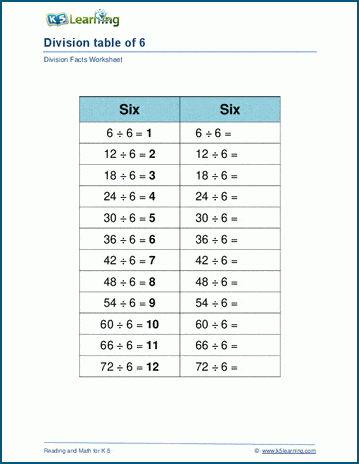## Mixed division facts worksheets

We make the next section of worksheets a bit more challenging by grouping dividing numbers by 2/3/4, 4/5/6, 7/8/9, 10/11/12. Each page starts with a division table and the worksheets follow. This is the table for 7/8/9: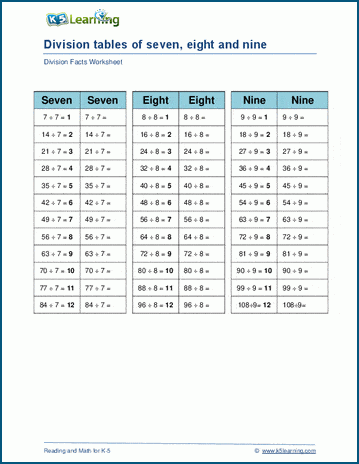## Division circle drills

Circle drills are a very effective and visual way to drill math facts. These circle drills practice division of 1-10 and 2-12. This is one of the 2-12 circle drills worksheets: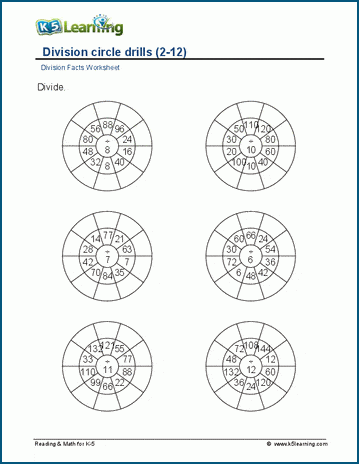## Missing dividends and divisors practice

The final section flips the script. Students work on missing dividends and divisors on these division facts worksheets. This worksheet covers the number 1-10.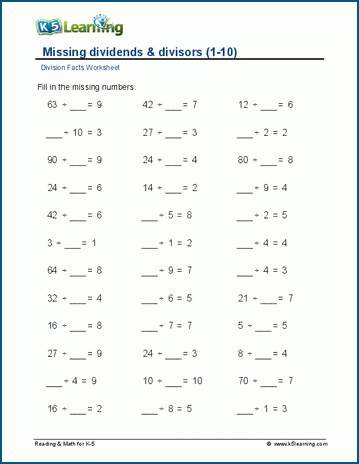Become a Member

This content is available to members only.